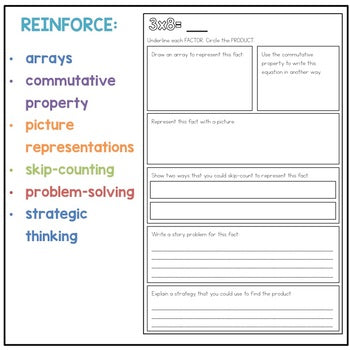# Daily Multiplication Practice# Daily Multiplication Practice

Regular price \$0.00
/
Be the first to leave a review.
• Grades: 3rd - 4th, Homeschool
• Pages: 2 pages
• Topics: Multiplication

#### Pairs well with

This is a two-page free sample of the bigger Multiplication Equation of the Day resource.

Equation of the Day is perfect as a daily Math Warm-Up or even as morning work.

This Multiplication Equation of the Day reinforces:

- the commutative property

- skip-counting to solve a multiplication equation

- multiplication strategies

- representing multiplication equations with arrays and pictures

- problem-solving

Ready to purchase the full Multiplication Equation of the Day resource? Find it HERE.

Looking for other Multiplication resources? You might be interested in:

Recently viewed